# Inverse Proportion Worksheet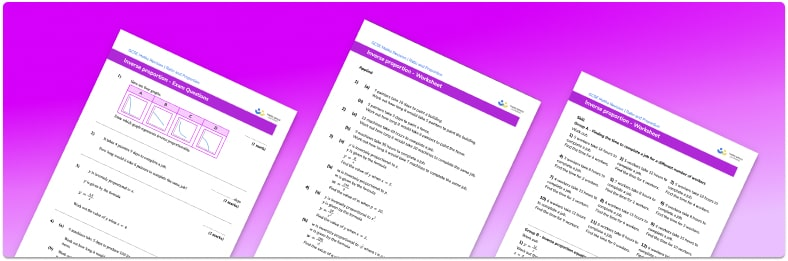• This field is for validation purposes and should be left unchanged.

You can unsubscribe at any time (each email we send will contain an easy way to unsubscribe). To find out more about how we use your data, see our privacy policy.

• Section 1 of the inverse proportion worksheet  contains 30 skills-based inverse proportion questions, in 3 groups to support differentiation
• Section 2 contains 3 applied inverse proportion questions with a mix of worded problems and deeper problem solving questions
• Section 3 contains 4 foundation and higher level GCSE exam style inverse proportion questions
• Answers and a mark scheme for all inverse proportion questions are provided
• Questions follow variation theory with plenty of opportunities for students to work independently at their own level
• All questions created by fully qualified expert secondary maths teachers
• Suitable for GCSE maths revision for AQA, OCR and Edexcel exam boards

### Inverse proportion at a glance

When two amounts are inversely proportional to each other, one amount increases as the other decreases. Another way of looking at this is if x is inversely proportional to y then x is proportional to 1 divided by y.

We see inversely proportional relationships in everyday life.  For example, the amount of time it takes to travel somewhere is inversely proportional to the speed at which we are travelling or the amount of time taken to complete a job is inversely proportional to the number of workers.

For more complicated relationships involving inverse proportion, we can find the constant of proportionality, k, and use this to write a formula connecting the two amounts. With y being inversely proportional to x, the value of k is equal to the product of x and y.

Inverse proportion is also known as indirect proportion, or inverse variation.

Looking forward, students can then progress to additional ratio and proportion worksheets, for example a ratio worksheet or a simplifying and equivalent ratios worksheet.

For more teaching and learning support on Ratio and Proportion our GCSE maths lessons provide step by step support for all GCSE maths concepts.

## Related worksheets

Proportion Worksheet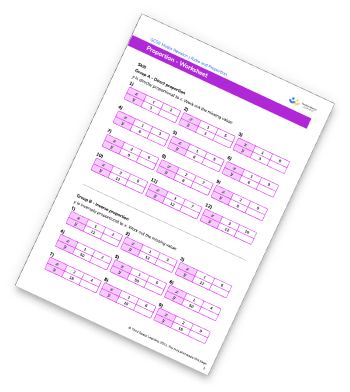Direct Proportion Worksheet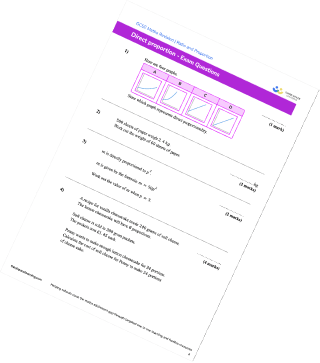Directly Proportional Graph/ Inversely Proportional Graph Worksheet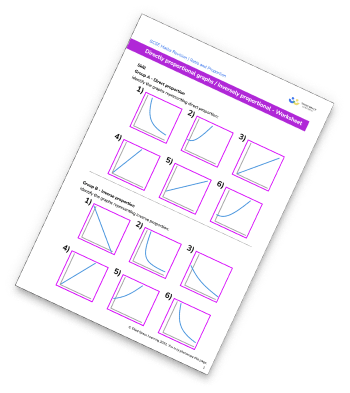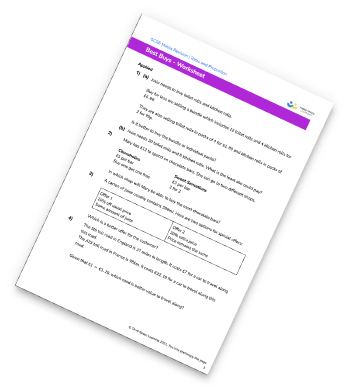## Do you have KS4 students who need more focused attention to succeed at GCSE?There will be students in your class who require individual attention to help them succeed in their maths GCSEs. In a class of 30, it’s not always easy to provide.

Help your students feel confident with exam-style questions and the strategies they’ll need to answer them correctly with our dedicated GCSE maths revision programme.

Lessons are selected to provide support where each student needs it most, and specially-trained GCSE maths tutors adapt the pitch and pace of each lesson. This ensures a personalised revision programme that raises grades and boosts confidence.

Find out more